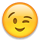Welcome to the Onshape forum! Ask questions and join in the discussions about everything Onshape.

First time visiting? Here are some places to start:
1. Looking for a certain topic? Check out the categories filter or use Search (upper right).
2. Need support? Ask a question to our Community Support category.
3. Please submit support tickets for bugs but you can request improvements in the Product Feedback category.
4. Be respectful, on topic and if you see a problem, Flag it.

If you would like to contact our Community Manager personally, feel free to send a private message or an email.

# Help with enclose function?

edited November 2021
Hello All,
I am trying to make a basic FeatureScript to improve my learning that generates RPG Dice (D4, D6, D8, D10, D12, D20).

I can successfully create planes for the sides of the D4 and wish to use an enclose operation to create the solid. What is the proper way to use enclose()? There is no documentation for opEnclose and I do not know how to reference the planes that I created earlier in the for loop.

Code snippet below:
```if(definition.DiceType == DiceType.d4)
{
const numPlanes = 4;
var d4_side = (20/16)*(definition.size);
var vertex1 = location - (d4_side/2)*vector(1,0,0) - (d4_side/(2*sqrt(3)))*vector(0,1,0);
var vertex2 = location + (d4_side/2)*vector(1,0,0) - (d4_side/(2*sqrt(3)))*vector(0,1,0);
var vertex3 = location + (d4_side/sqrt(3))*vector(0,1,0);
var vertex4 = location + ((d4_side*sqrt(3))/(2))*vector(0,0,1);
var points = [vertex1, vertex2, vertex3, vertex4];
//make 4 planes
var planes = "";
var face = [];
for(var i = 0;i<numPlanes;i+=1)
{
face = [points[i],points[(i+1)%numPlanes],points[(i+2)%numPlanes]];
planeCalc(context, id+("planeCalc"~i), face, "plane"~i);
//planes = [planes + ("plane"~i)];
}

//operation for making enclosed part from planes?
/*enclose(context, id+"enclose1", {
"entities": //WHAT TO PUT HERE? How do I properly ref the created planes?
});
*/
}

function planeCalc(context is Context, id is Id, face is array, name is string) //FUNCTION DEFINITION HERE
{
//Calculate Plane through 3+ points
var total = vector(0,0,0)*millimeter;
var numPoints = size(face);
for (var item in face)
{
total += item;
}
println(total);
var a = face;
var b = face;
var c = face;
var _origin = total/numPoints; //calculates center point of face
var _normal = cross((c-a), (b-a)); //uses 3 of the points on a face to make the plane
opPlane(context, id + name, {
"plane" : plane(_origin, _normal),
"width" : 250 * millimeter,
"height" : 250 * millimeter
});
}```

SOLVED:  "entities" : qCreatedBy(planeId, EntityType.BODY) <- This goes in enclose!

• Member, Onshape Employees Posts: 74• There's a few features without documentation unfortunately (`extend` and `offset`, for example). When in doubt, check out the source code: enclose & opEnclose. Takes a few extra minutes, but usually works